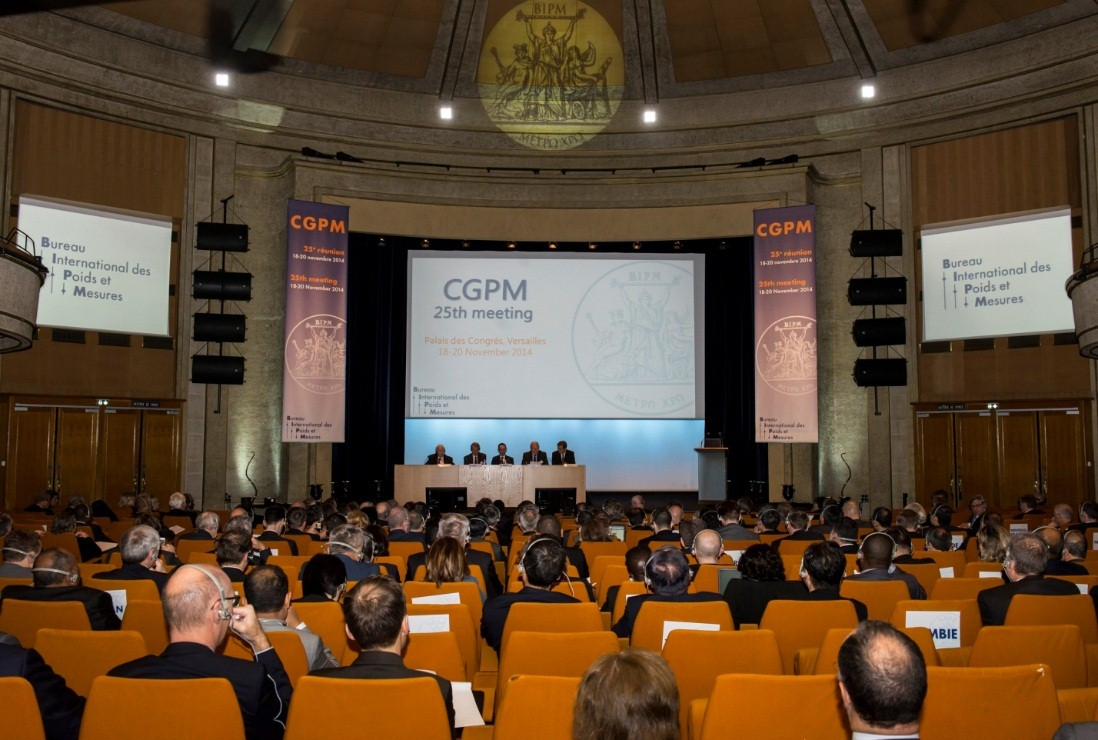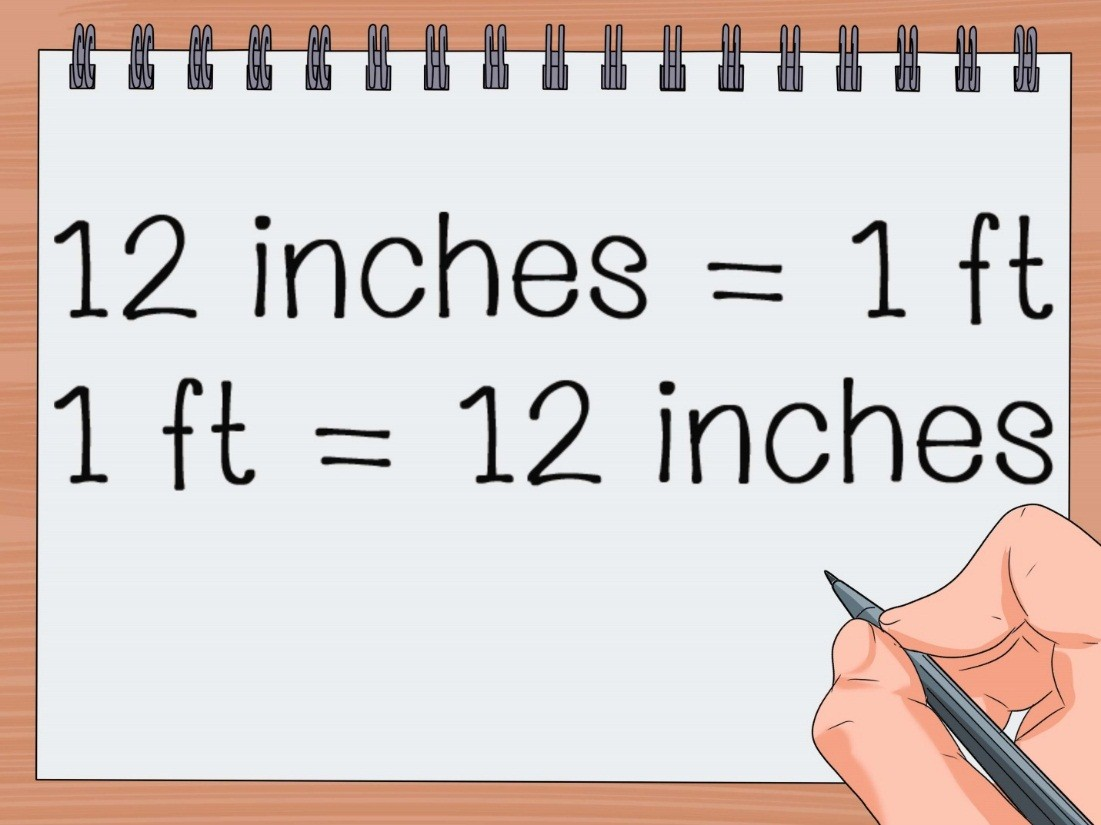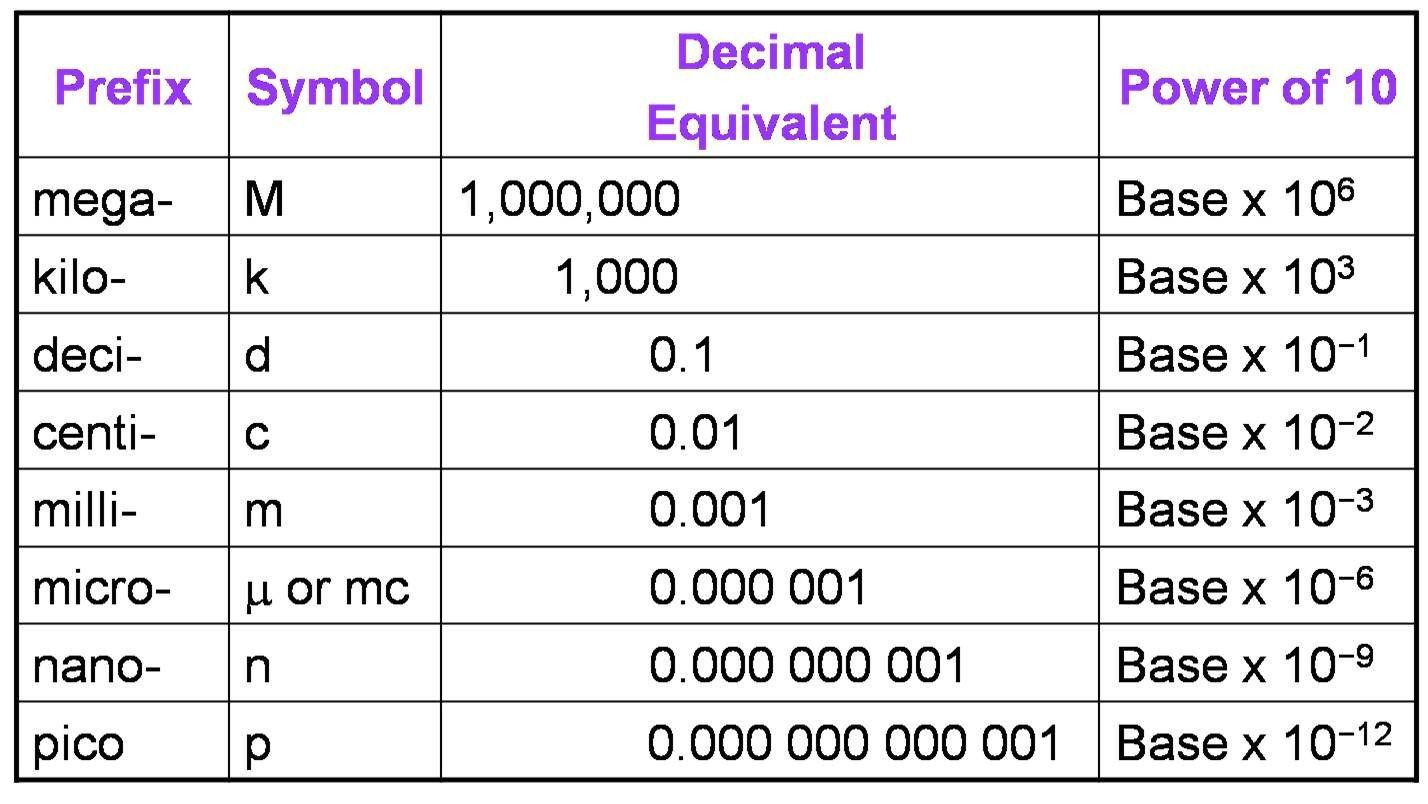# The International System of Units

## System of Units

Every quantity in physical world requires a unit to explain or define it. It is because of units only; the physical world is classified so well. Imagine what the condition of Earth would be if there is no unit. To be precise, we won’t be able to calculate things which will deteriorate the business markets. Adding further we won’t be able to define quantities, which would end up creating a lot of daily problems to all of us. To avoid so many confusions and problems physicist defined unit.

Now, the question arises are units enough to define quantities? The answer is partial yes as the world has many divisions, it has many languages and it is not possible to define a certain unit for the entire universe.

Example: A farmer supplies food grain in America, through a transportation system. The weight of 1 sack of grain is around 60 kg and there are around 1000 sacks. How much money will the farmer get from America, if the rate of food grain in America is 4\$ per pound?

The problem is pretty simple. The physical quantity involved here is weight. In India, the standard unit of weight is kilograms so the total weight of sacks will be 60,000 kilograms. In America, the unit of weight is pound so we will convert kilograms into pounds and calculate the overall rate of food grain as the cost of 1000 pounds grain is 4\$.

1 kilogram = 2.20 pounds

Then the weight of 60,000 kilograms in pounds will be = 2.20 × 60,000 = 1, 32,000 pounds

Cost of 1000 pounds food grain = 4\$

Cost of 1, 32,000 pounds food grain will be = 4/1000 × 1, 32,000 = 528\$

Thus, the total amount farmer will make will be 528 dollars.

The System of Unit is defined as a set of units involved in arithmetic operations of various physical quantities with the help of conversion factors. It further helps in conveying information from one region to another in terms of units which makes business and relations much easier.

## Benefits of having a System of Units

The system of Units has following benefits in everyday life situations. They are as follows:

• Easier to learn and solve conversion problems

• Simplifies physical quantities

• System of units is used to do measurement tasks internationally as it has interchangeability

• Convenient to use and practice

## Types of System of Units

There are three types of System of Units which we put into use for physical world to make measurements easier and reliable. The types of system of units are:

• International System of Units or M.K.S

• F.P.S system

• C.G.S system

### International System of Units or S.I.Image 2: CGPM held its 25th meeting to fix several units.

S.I or International System of Units was founded in 1971 by CGPM committee. The system is widely used all over the world and is also known as Metric System if units. The standard units of fundamental and derived quantities are discovered with the help of an international system of units. It is also known as the M.K.S System of Units. The full form of M.K.S is Meter, Kilogram and Second, which are standard units of length (meter), mass (kilogram) and time (second) respectively. The system has given recognition to several quantities globally. The table below depicts seven fundamental quantities and their standard unit.

 Quantity Unit Symbol Mass Kilogram kg Time Second s Temperature Kelvin K Electric Current Ampere A Luminous Intensity Candela cd Length Meter m Amount of Substance Mole mol

There are two additional units for measuring angles they are:

• Steradian for solid angles denoted by symbol sr

### F.P.SImage 3: A conversion factor to convert foot to inches.

The F.P.S or foot pound second system is system of units based on three fundamental units that is, foot for length, pound for weight and second for time. The system was used until 20th century and is still used in some parts of the world. The system is well acquainted with technical English publications. It is also called Stroud System, named after William Stroud who popularized this system.

### C.G.SImage 4: C.G.S is another variant of metric system of units.

The C.G.S or centimeter-gram-second system is a system of units based on three fundamental units taht is, centimeter for length, gram for weight and second for time. It is another variant standard S.I system. In most measurement cases we use the standard system of units and the C.G.S system is used only in some dominant systems. It was used in earlier times. But when people found it confusing and irrelevant as the length of rooms and buildings in centimeters came out to be very large and it was very difficult to remember numbers in this system, so scientists and physicists preferred to refer the M.K.S system as the standard system of units.

## S.I PrefixesImage 5: We use some S.I prefixes to make fundamental quantities convenient for use

The magnitude of physical quantities deviates over a wide range and generally, we write very large or small quantities in the form a × 10b. Some examples based on this form are:

• Separation between two protons inside a nucleus is about 10-15m

• Mass of electron is 9.1 × 10-31 kg

• Mass of galaxy is around 2.2 × 1041 kg

We S.I prefixes in order to represent quantities conveniently and also name them with standard prefixes. The table given below depicts various prefixes, their symbols, and power of 10.

 Prefix Power of 10 Symbol exa 18 E peta 15 P tera 12 T giga 9 G mega 6 M kilo 3 k hecto 2 h deca 1 da deci -1 d centi -2 c milli -3 m micro -6 μ nano -9 n pico -12 p femto -15 f

Watch this Video for more reference

The international system of Units### Course Features

• Video Lectures
• Revision Notes
• Previous Year Papers
• Mind Map
• Study Planner
• NCERT Solutions
• Discussion Forum
• Test paper with Video Solution